# Wave Particle Duality Principle

With the development of Photoelectric effect, Crompton’s effect and Bohr’s model of atom, the idea of light or in fact radiations in general, being composed of particles or discrete Quanta was gaining wide popularity.
However, the very established Huygen’s Principle and the results of Young’s double slit experiments made it very clear that light was wave and not a flow particles.The striking interference pattern observed by passing light through double slits was definitely a result of the wave nature of light. This again gave rise to the controversy of nature of light. In 1704 Newton had also suggested the particle nature of light by his corpuscular theory.

Neither of the two theories were adequate enough to explain all the phenomena associated with light. Thus scientists began to conclude that light has both wave and particle nature. In 1924, a French physicist, Louis de Broglie came up with a theory. He suggested that all particles in this universe is associated with wave nature also, i.e. everything in this world be it a small photon or a giant elephant, everything has an associated wave with itself, it is a different matter that the wave nature is noticeable or not. He assigned a wavelength to each matter with mass m and momentum p asWhere, h is Planck constant and p = mv, v is velocity of the body.

Thus due the huge mass of an elephant it has a very significant momentum and hence a very small wavelength, which we are unable to notice. However small particles such as electrons, etc. have very small mass and hence very noticeable wavelength or wave nature. This theory of de Broglie also helps us to explain the discrete existence of orbits in Bohr’s model of atom. An electron will exist in an orbit if its length is equal to integral multiple of its natural wavelength, if it is unable to complete its wavelength then that orbit will not exist.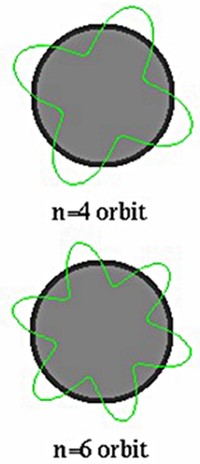Further developments by Davisson and Germer of electron diffraction from a crystal and a similar interference pattern obtained after bombarding a double slit with electrons had strengthen de Broglie’s matter wave theory or the wave particle duality theory.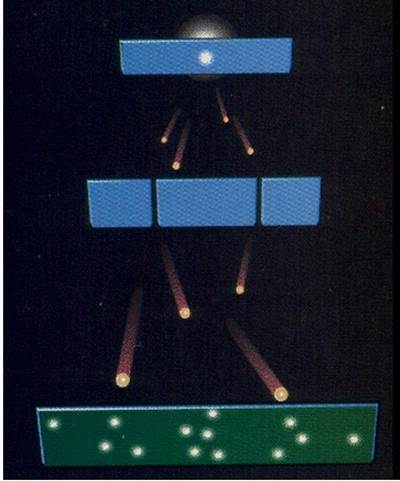## Compton Effect

In the photoelectric effect, the light strikes on a metal in the form of beam of particles called photons. The energy of one photon contributes the work function energy of one electron as well as provides the kinetic energy to that emitted electron. These photons are the particle like behavior of light wave. Sir Albert Einstein proposed that light is the collective effect of huge number of energy packets called photon where each photon contains energy of hf. Where h is the Planck constant and f is the frequency of the light. This is a particle like behavior of light wave. The particle like behavior of light-wave or other electromagnetic wave can be explained by Compton effect.

In this experiment, one x ray beam of frequency fo and wavelength λo was incident on an electron. After hitting the electron by incident x-ray it is found that the electron and incident x-ray both are scattered into two different angles with respect to the axis of incident x-ray. This collision obeys the energy conversation principle just like collision of Newtonian’s particles. It was found that after the collision the electron gets accelerated in a particular direction and the incident x-ray is diffracted in another direction and it was also observed that diffracted ray has a different frequency and wavelength than the incident x-ray. As the energy of the photon varies with frequency it can be concluded that the incident x-ray losses an energy during collisions and the frequency of the diffracted ray is always less than that of the incident x-ray. This lost energy of x-ray photon contributes the kinetic energy for the movement of the electron. This collision of x-ray or its photon and electron is just like to Newtonian’s particles such as Billboard balls.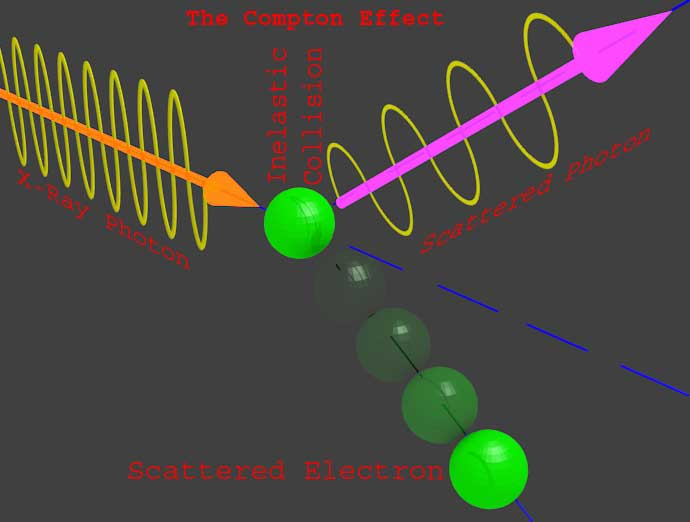The energy of photon is given by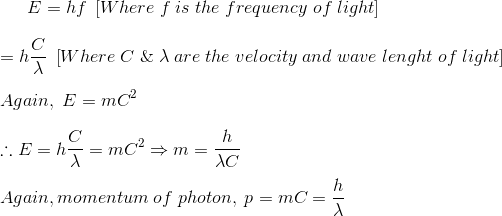Therefore the momentum of the photon can be proved as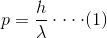Which can be written as,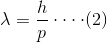From equation (1) it can be concluded that a electromagnetic wave with wavelength λ will have the photon with momentum p.
From equation (2) it can be concluded that a particle with momentum p is associated with wavelength λ. That means wave has particle like characteristics, the particle on movement also exhibits wave like behaviour.

As we already said, this conclusion was first drawn by De Broglie and hence this is known as De Broglie hypothesis. As the wavelength of the moving particle is expressed asWhere, p is the momentum, h is Planck constant and wavelength λ is referred as De Broglie’s wavelength. De Broglie explained that as the electrons orbit around the nucleus it will also have the wave like behaviour along with its particle like characteristics.

## Divission and Germer Experiment

The wave nature of electron can be proved and established in many different ways but most popular experiment is Divission and Germer in the year of 1927. In this experiment they used a beam of accelerated electrons which normally strikes on the surface of a nickel block. They observed the pattern of scattered electrons after striking on the nickel block. They used an electron density monitor for this purpose. Although it was expected that the electron should be scattered after collision in different angle with respect to the axis of the incident electron beam but in the actual experiment it was found that the density of scattered electrons was more at particular angles than other. This angular distribution of the scattered electrons is very similar to an interference that of light diffraction. Hence this experiment clearly shows the existence of wave particle duality of electrons. The same principle can be applied to the proton and neutrons too.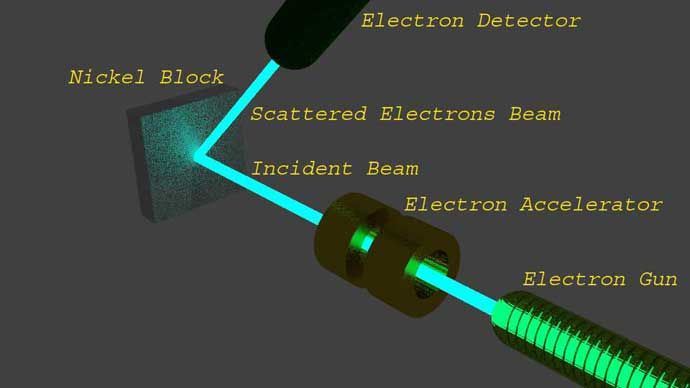Want To Learn Faster? 🎓
Get electrical articles delivered to your inbox every week.
No credit card required—it’s 100% free.